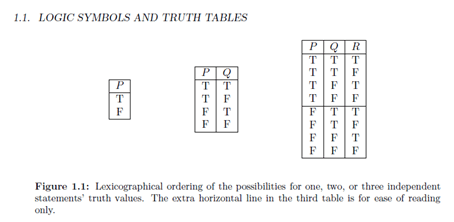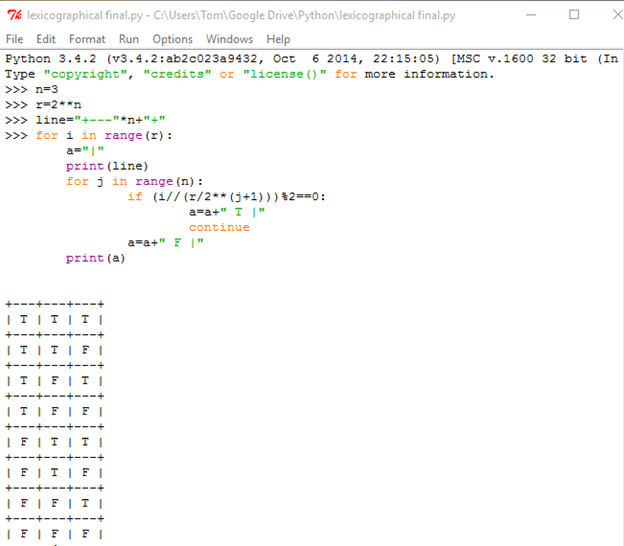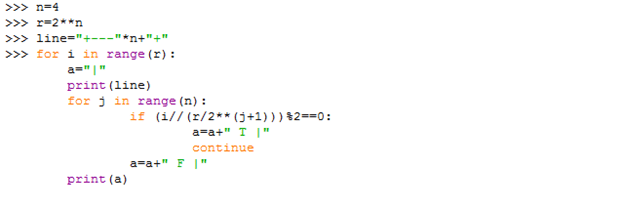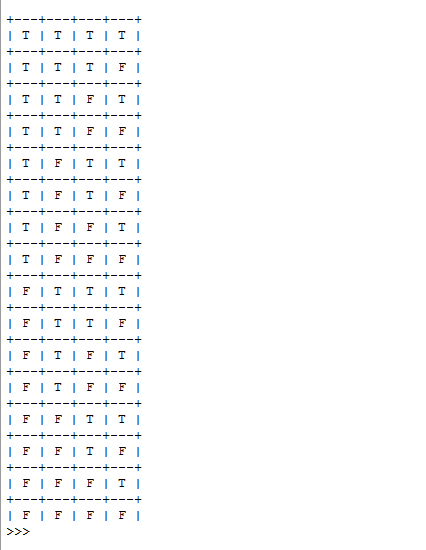# MATH318 Project 1 Solved

25.00 \$ 12.50 \$

Category:

## DescriptionThe assignment is to write a python program that will take the number of component statements and produce a lexicographical truth table for that number of statements.  Test your program with three and then 4 statements.    Because this is the first programing assignment I have given an example solution below.   If you use it you must change the names of the variables, reenter it, show it works.  And then submit a short explanation of why it works.

Do this in idle and send me your file.

If you need to download python you can do it here.  I am using the Python  3.4 idle version.

If you need a reference for python here is a good place to start.

Python tutorial

https://docs.python.org/2/tutorial/introduction.html

There is a partial explanation below the code.   The code is from the idle environment and I am fine with that.Each column repeats t or f some number of times

Left column alternates t and f

The one to its right repeats t twice then f twice

r/2**(j+1)   will give the number to times t repeats in the j column from the left.  That is j=0 is the right most column.   In the case above with 4 statements r=2**4=16  is j=0 then 16/2**1=8.

// is floor division so if the row number  floor divided by the numbers of repeats is even or zero we have a T.  Otherwise F.